## ODDFPRICE calculation differences between EXCEL and XLeratorDB

Jul 14

Written by: Charles Flock
7/14/2009 6:43 PMIf you use the EXCEL ODDFPRICE to calculate the price of a bond with an odd first coupon period, you should read this article to find out when those calculations are incorrect. Specifically, by using the EXCEL PRICE function you will discover the following three problems with the ODDFPRICE function...
If you use the EXCEL ODDFPRICE to calculate the price of a bond with an odd first coupon period, you should read this article to find out when those calculations are incorrect. Specifically, by using the EXCEL PRICE function you will discover the following three problems with the ODDFPRICE function:
1.       The day count conventions are applied inconsistently between the PRICE function and the ODDFRPICE function;
2.       The calculation of ODDFPRICE may be off by one full coupon period where there is a long odd coupon period and the maturity date is the last day of the month; and
3.       The calculation of coupon dates in the quasi-coupon periods is wrong where the maturity date is the 31st of the month and the first coupon date is not.

Background

The ODDFPRICE function is used to calculate the price, given the yield, of a bond with an odd first coupon period. The term ‘odd first coupon’ means that the issue date (or the dated date, if you prefer) of the bond does not fall on a ‘regular’ coupon date and the first coupon payment/period will be different than all the regular coupon payments/periods. This ‘odd’ first coupon payment can change the overall price calculation significantly because of both the value of the first coupon payment and the accrued interest it generates over the life of the bond.
Many bonds have this characteristic and these odd coupons can either be ‘long’ or ‘short’. A long odd coupon period means that the period from the issue date of the bond to the first coupon date is longer than the regular period. A short odd coupon means that the period from the issue date of the bond to the first coupon date is shorter than the regular period. While it is theoretically possible that the odd period could be longer than two regular coupon periods, it is highly unusual for this to be the case in the real world.
Once the bond reaches the first coupon date, it is then priced like any other bond as all the remaining coupons are regular. The regular coupon payments are calculated in a quite straightforward manner using the PRICE function. They are simply the par value multiplied by the coupon rate divided by the number of coupon payments in a year. In EXCEL, the par value is assumed to be 100 and the number of coupon payments in a year can either be 1, 2 or 4.
There are 2 different formulae for calculating the ODDFPRICE. The first, for odd short first coupons is: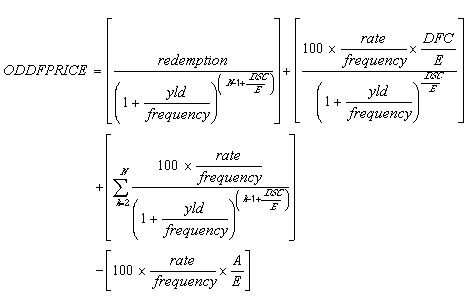And the second, for an odd long first coupon is: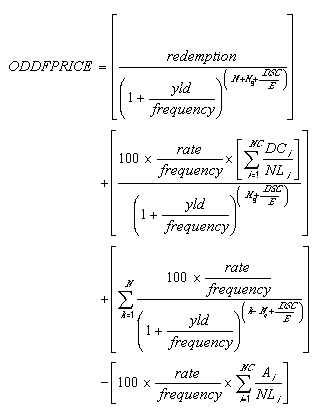While these look quite complicated, the following explanation should simplify things. In both examples let the first term (let’s call it term1) represents the discounted value of the redemption value of the security. The second term (term2) is the discounted value of the odd coupon interest. The third term (term3) is the discounted value of regular coupons. The final term (term4) is the accrued interest.

Methodology

Now, if we assume that the interest rate is zero, then terms 2, 3 and 4 are equal to zero and the price, given the yield, of the bond is simply the discounted value of the cash flows. This then lets us calculate the value of the exponent in the first term. Let’s look at an example.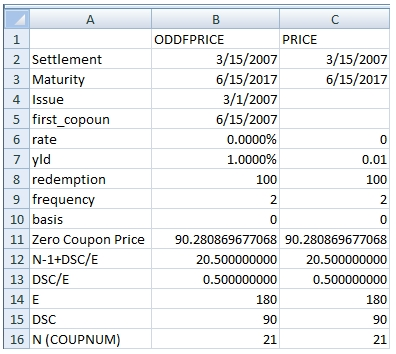Here we have put together an example of a bond with a short odd coupon period. Column A simply contains the names of key variables. Column B contains data used in the ODDFPRICE function. Column C contains data used in the PRICE function, copied from column C. Rows 2 through 10 contain the data. Note that the rate is zero.
In cells B11 and C11 we have entered the ODDFPRICE and the PRICE functions. Then using the LOG function, we can calculate the N-1+DSC/E value which was used by the functions. In B16 and C16 we have used the COUPNUM function to calculated N. It than becomes straightforward to calculated the DSC/E fraction. Since E is the number of days in quasi-coupon period, the COUPDAYS function can be used. From there, it’s a simple multiplication step to get the value for DSC. Here is a snapshot of the spreadsheet with the formulae: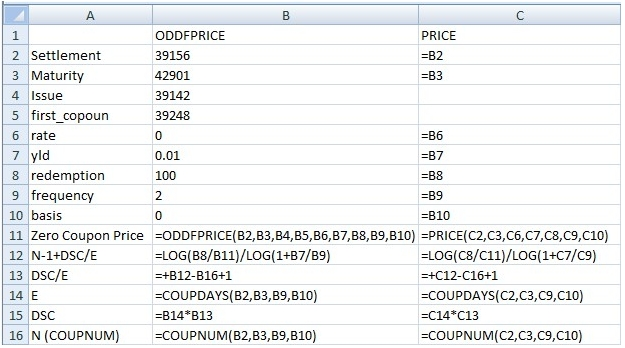This gives us the capability to compare the consistency of the calculations between the PRICE function and the ODDFPRICE function. We can now use this capability to analyze the consistency of the day count conventions.

Basis Problems

Let’s see what happens when we change the settlement date to 5/31/2007: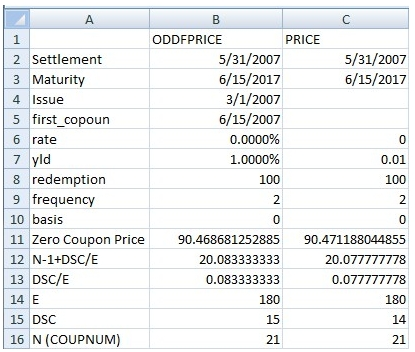As you can see, B11 and B12 now have different values. Using the formulae that we entered, it is quite plain that ODDFPRICE calculated the DSC as 15 days and PRICE calculated the DSC as 14 days.
This problem clearly arises as a result of how the 31st of the month is treated when the basis is 0. Testing against other bond calculators like FICALC, it seems that the implementation in the PRICE function gives the desired result.

Quasi-coupon problems

Let’s look at a different problem; calculating the number of coupons in the quasi-coupon period when there is an odd long first coupon. Let’s take a bond with an odd long first coupon and let’s set the basis equal to 1. Of course, when we do that, we have to modify the formula in cells B14 and B15 because of the inconsistency between the COUPDAYS function and the PRICE function (
see my June 30, 2009 blog on this subject). So now the formula looks like this:
IF(C10=1,COUPNCD(C2,C3,C9,C10)-COUPPCD(C2,C3,C9,C10),COUPDAYS(C2,C3,C9,C10))
Here’s the example: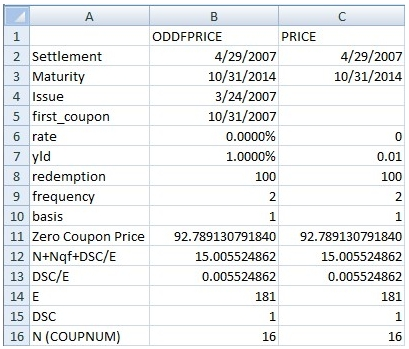As you can see, both calculations return a value for DSC of 1, meaning that there is one day from the settlement date until the next coupon date. This implies that the coupon date is 4/30/2007, but we know from the data that the first coupon date is 10/31/2007, so this date is referred to as the quasi-coupon date. And the label for the exponent in A11 has been changed to N+Nqf+DEC/E where Nqf is the number of quasi-coupons, which is defined as the number of whole quasi-coupon periods between settlement date and first coupon.
Now, let’s see what happens when we advance the settlement date to 5/1/2007.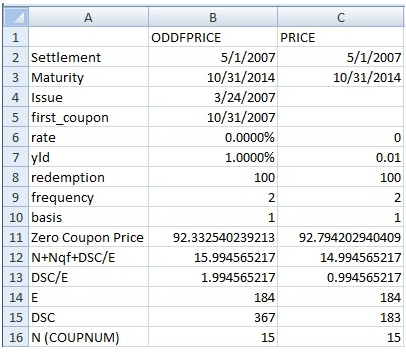As we can plainly see by looking at cells B12 and C12, there is a difference of one whole coupon between the two calculations. This difference persists throughout most of the quasi-coupon period, though there are some days when it is correct. We have checked this calculation against numerous bond calculators and there is no doubt that the EXCEL calculation is inconsistent with industry practice as well as being inconsistent with PRICE function.
Since this is a zero-coupon bond, it looks like the difference is small. This is no small problem, however. If we had a bond that was paying interest, the difference could be 300 or 400 basis points. On a million dollars in bonds, that would equate to 30 or 40 thousand dollars.
Holding the issue, first_coupon, and maturity date constant and varying the settlement date from 3/25/2007 to 10/30/2007, we found that the only dates for which the EXCEL ODDFPRICE calculation was correct were 3/31/2007 through 4/30/2007, inclusive. The calculation for all other settlement dates was incorrect.
If we change the first coupon date to 11/30/2007 and the maturity date to 11/30/2014, we found that the only dates where the EXCEL ODDFPRICE calculation was correct were 4/30/2007 through 5/31/2007, inclusive.
Interestingly enough, when we set the first coupon date to 11/15/2007 and the maturity date to 11/15/2014, all the calculations for all the settlement dates are correct.
We found this problem to be pervasive across day count methods. In fact, it is an even bigger problem with day count methods 2 and 3, but since there are very few instruments that trade this way, we have ignored those at this time.

Calculation of quasi-coupon dates

Let’s look at the final problem that we discovered, that the calculation of coupon dates in the quasi-coupon periods where the maturity date is the 31st of the month and the first coupon date is not, is wrong. This problem really only manifests itself when the basis is 1, 2, or 3. We will focus on the basis of 1.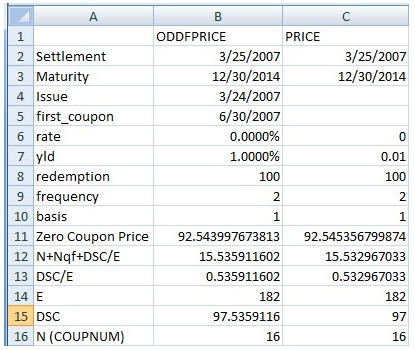We have a maturity date of 12/30/2014, a first coupon date of 6/30/2007 and a settlement date of 3/25/2007. As you can see in cell C15, using the price function, we calculate a DSC of 97 days. Using the ODDFPRICE function, we calculate a DSC of 97.5359116. If was assume that 97 days is the right answer, we can quickly determine that 97/181 gives us the result that is in B15, so it seems that the ODDFPRICE function has a value of 181 for E and the PRICE function has a value of 182 for E.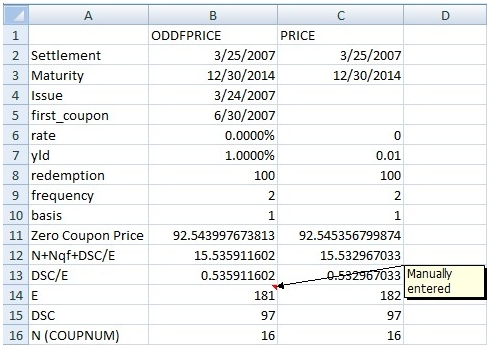We can infer that the ODDFPRICE function thinks that the quasi-coupon period begins on 12/31/2006 and that PRICE thinks that the quasi-coupon date is 12/30/2006. One way that this could happen is if the ODDFPRICE function is calculating the quasi-coupon periods using the first-coupon date rather than the maturity date of the security. The way ODDFPRICE securities work, the first coupon date must be a regularly scheduled coupon date (that’s why we can compare the ODDFPRICE function to the PRICE function this way). However, using the COUPPCD function, we can see that we will get different results, depending on whether the maturity date or the first coupon date is used: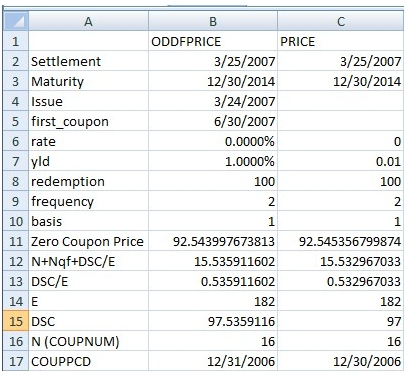In cell B17 we have calculated the previous coupon date using the first coupon date and in cell D17 we have calculated the previous coupon date using the maturity date.
Checking against other bond calculators it appears that the industry practice is to calculate the coupon dates using the maturity date and that the EXCEL calculation is inconsistent with that practice.

Conclusion

We have described three different problems with the EXCEL ODDFPRICE calculation, each of which we have analyzed using other EXCEL functions. We have implemented the ODDFPRICE function to be consistent with the PRICE function and with industry practice, so it is possible that your results could be different from EXCEL.

Tags:
Categories:
Location: BlogsThe WestClinTech Blog

## Search Blogs

 KeywordsPhrase

## Blog Archives

Archive
 < September 2023 >
SunMonTueWedThuFriSat
272829303112
3456789
10111213141516
17181920212223
24252627282930
1234567
Monthly
Go

### SupportCopyright 2008-2023 Westclintech LLC         Privacy Policy        Terms of Service